﻿ Computational Analysis of Curved Beams - In and Out-of-Plane VibrationsPublications are Open
Access in this journal
Article Versions
Export Article
• Normal Style
• MLA Style
• APA Style
• Chicago Style
Research Article
Open Access Peer-reviewed

Computational Analysis of Curved Beams - In and Out-of-Plane Vibrations

Raposo F.O.
Journal of Mechanical Design and Vibration. 2021, 9(1), 1-12. DOI: 10.12691/jmdv-9-1-1
Received March 04, 2021; Revised April 07, 2021; Accepted April 16, 2021

Abstract

In the present work are shown results obtained from a computational model for the dynamic analysis of planed curved beams with constant cross-section and curve radius, based on the exact solution of the differential equilibrium equations of a beam element. This model allows the estimation of the forced harmonic motion, both in-plane and out-of-plane of curvature on a free-free support condition. The input data of the model is the geometry section of the beam, material properties, type of motion, consideration of the shear stiffness, and rotatory inertia effects. The output result is possible either in a frequency response of a fixed parameter curved beam or a plot representation of the natural frequencies curves for an opening angle range, from an approximated straight beam to a closed opened ring. Each of those curves is defined as family of modes and performed on present work. The objective of this work is to clarify the general behaviour of curved beams with the mentioned conditions by using an accurate model showing results as a reference for the scientific community. The model was validated by testing and comparing results with three beam specimens previously studied in other publications. The biggest aim is also to present new results and hypothesis, contesting other authors regarding the natural frequencies of curved beams.

1. Introduction

The subject of dynamic analysis of curved beams is a delicate one, that involves complex equations on the calculation of its frequency response function (FRF). Given this complexity, it is a great value to change the paradigm of this theme to a computational scope where there has been work previously developed. The development of this work is based on a philosophy of adapting an analytical model to a computational context, by implementing it computationally, increasing the efficiency of dynamic analysis studies.

Over time, the name of Hoppe 1 has been cited in immense studies that deal with the dynamic study of curved beams and rings because he was the researcher with one of the oldest publications on this topic, who deduced in 1871 the expression that allows obtaining values of natural frequencies for thin circular rings, for in-plane motion, disregarding the extension of the central line and the effects of shear deformation and rotatory inertia.

Years later, Love 2 developed a remarkable theory about elasticity in his book published in 1944 that allowed the knowledge of the natural frequency values for thin circular rings, applicable to both types of motion, inside and outside the plane of curvature.

The authors Seidel and Erdélyi 3 tried in 1964 to solve the recurrent issue of the neglection of the referred effects for the in-plane motion of circular rings by counting the energies associated to flexion, shear deformation, translation and rotation of rings. Afterwards, the simplification of not including the energy associated with the extension of the central line was made due to the lack of computational resources.

Rao and Sundararajan 4 obtained a dynamic equilibrium equation for in-plane motion of rings, taking into account the effects due to shear deformation and rotatory inertia, making the study separately from the inclusion of one effect and the other, concluding that the effect due to the shear deformation would have a predominance rather than the rotatory inertia leading to greater proximity with the experimental values. Some authors have investigated vibrations in circular rings, but without this step. Consequently, this work done in 1969 has proved to be a great asset in the area of circular rings.

It was a fact that there were theories that supported the calculation of natural frequencies for circular rings and there were later authors who calculated natural frequencies within boundary conditions for in and out-of-plane, but commonly dismissing the mentioned effects.

Timoshenko et al. 5 left his great mark in 1921, by showing that the deviations caused by the non-consideration of shear deformation in straight beams, could be tremendous, incurring great errors in the calculation of natural frequencies, for this case.

Wang et al. 6 in 1984 used an analytical process to obtain the dynamic stiffness matrix of an out-of-plane vibrating curved beam, referring to the accounting of the effects of rotatory inertia and shear deformation. However, the inclusion of rotatory inertia is not complete since this term, associated with the rotation (torsion) of the cross-section, is neglected in the equilibrium equations, as shown by Urgueira 7.

On that way, Urgueira 7 developed the study that supports the present work, dealing with the development of an analytical model of dynamic analysis of circular curved beams of constant cross section, through the exact solution of the differential equations, which contemplates the motion inside and outside of the curvature plane including the shear deformation, the rotatory inertia, and the extension of the central line axially regarding the in-plane vibrations.

Later in 1987, Montalvão e Silva and Urgueira 8 published a work where the comparison between Wang et al. 6 model for the out-of-plane motion was made by using the same beam reference, including the term responsible for the rotation of the section. These authors pushed the subject towards the question of what the curves containing natural frequencies would be like as a function of the opening angle for a beam of constant length. The major conclusion of the publication was that the rotary inertia associated with coupling with torsional effects couldn’t be neglected, by comparison of results.

A few years later in 1998, Tufekçi and Arpaci 9 also determined the exact solution of differential equations for arcs on free-free conditions and in-plane vibrations. They have studied in particular several boundary conditions applied to this motion by comparing the natural frequencies obtained with and without the presence of the shear deformation, rotatory inertia and extension of the central line.

The same researcher Tufekçi and Dogruer 10 elaborated another work in 2002, whose exact solution is then determined for free-free out of-plane vibrations, particularly for curved beams of rectangular section. On that work, the effects of deformation due to shear and rotatory inertia are accounted for in this motion. The solution was determined by using the initial values method, depending on the boundary conditions. They used the experimental data and the same beam reference in 7 making a general comparison between their model, Wang et al. 6, and Urgueira 7, having the experimental reference of this last researcher.

The curves that contain the referred resonance frequencies defined here as mode of families were not deeply investigated quantitatively by researchers according to the bibliographic research carried out, possibly due to the enormous complexity of humanly performing the analytical calculation of frequency responses for a given opening angle, moreover searching resonance points repeating this cycle times and times in order to have a reasonable representation of the curves that contain the families of modes.

The objective of this work is to present a new paradigm on the curved beams resonance frequencies evolution with its curvature using a computational tool developed based on the exact solution for free-free vibrations previously developed analytically. The quantitative results obtained allow to confirm and/or contest results from authors who have previously, investigated the dynamic behaviour in terms of possible modal properties. Those curves defined here as families of modes are sustained on the natural frequencies which are calculated from the frequency response function. To validate the families of modes, it is mandatory to validate the resonance frequencies. Consequently, the structure of this study begins with three beam specimens used by authors in previous publications whose natural frequencies are taken here as a reference to test the computational results. The set of curves that represents the group of the first four (families of modes) natural frequencies are presented and compared with the ones from specialized authors on the subject. Besides the new achievements presented on this work, the aim of this study is also to provide realistic results (resonance frequencies and FRF) for further studies regarding curved beams, rings, and arches.

The current article comprises the following sections: introduction, analytical formulation, computational analysis, the families of modes concept, results and comparisons, and conclusions. After the introduction, the analytical basis of the computational is shortly presented in the section analytical formulation. The section computational analysis presents the curved beams specimens selected to test the consistency of the calculations executed by the computational model such as the frequency response functions and natural frequencies. The families of modes concept section presents the plots of the mentioned curves and those are later compared with ones from other articles. The final interpretation regarding the results in the present work is done focusing on the improvements done and potential topics to investigate for authors.

2. Analytical Formulation

The beam is generally defined as an elongated structural element generated by its cross-section, intended for the application of loads. In this context, the curved beam is characterized by its cross-sectional height Alt, radial thickness Esp, and the constant radius of curvature R along its opening angle α, as well as by the homogeneous material that joins the two ends as defined in the works of Urgueira 7 and Raposo 13 (Fig. 1).

• Figure 1. Geometric parameters of a curved beam

Consider a curved beam, the central line of which joins the centroid of all cross-sections, is contained in a plane called the Curvature Plane.

The study was confined to small deformations in curved beams whose length is larger when compared to the dimensions of the cross-section, i.e. the beam is considered slender. It turns out that the beam has six degrees of freedom at all points of its central line, between its opposite ends along all the cross-sections. This study is also particularized for curved beams whose cross-sections are symmetrical in relation to the axes x and y, where the directions of the local axis system coincide with the main directions of axial, shear, bending, and torsion deformations. As can be illustrated, Fig. 2 represents the local axis system applied to the two ends of the curved beam with the possible displacements and rotations of green and blue colour respectively.

• Figure 2. Curved beam displacements and rotations
2.1. Equilibrium Equations

The motion of the curved beam, as a three-dimensional space body, is characterized by the composition of three translations plus three rotations. According to the ordered axes, and this motion can be divided into the study of two types of motion, whose equations are shown in the sections 2.1.1 and 2.1.2.

2.1.1. In-Plane Vibrations

In Fig. 3 is represented a beam element in dynamic equilibrium, which indicates the normal stress N, the shear stressthe bending momentas well as the corresponding displacements w, u, and the rotation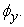• Figure 3. Degrees of freedom and stresses for in-plane vibrations

This type of motion can be represented in a two-dimensional plane in which radial u and tangential w displacements occur accompanied by the rotation of the cross-sectionThe differential equations of second order for this case were studied in the work Urgueira 7, being the equilibrium equations that deal this motion, the following: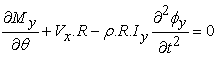(2.1.1a)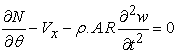(2.1.1b)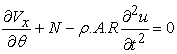(2.1.1c)

The FRF can be represented by one of the 36 elements in the receptance matrix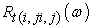for a certain range of frequencies (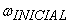towith a step). The relationship between displacements and stresses is given by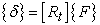with the arrangement in eq, (2.1.1d):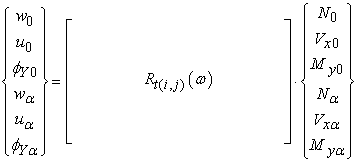(2.1.1d)

2.1.2. Out-of-Plane Vibrations

In Fig. 4 is represented a beam element in dynamic equilibrium, which indicates the bending moment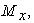the torsion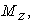the shear stress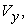as well the corresponding rotationsand the displacement v.

• Figure 4. Degrees of freedom and stresses for out-of-plane vibrations

This type of non-planar motion can be represented three-dimensionally since there are displacements on three planes in which rotations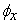and torsionof the section occur, accompanied by translation v perpendicular to the plane of curvature.

The differential equations of second order for this case were studied in the work Urgueira 7, being the equilibrium equations that deal this motion, the following:(2.1.2a)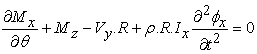(2.1.2b)(2.1.2c)

The FRF can be represented by one of the 36 elements in the receptance matrixfor a certain range of frequencies (to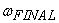with a step). The relationship between displacements and stresses is given bywith the arrangement in eq. (2.1.2d):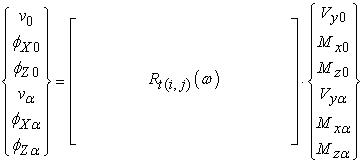(2.1.2d)

3. Computational Analysis

3.1. Reference Specimens

After the development of the computational model, rigorous tests were carried out on the values given by the program. The validation of the computational model was evaluated, in a first phase, with experimental data from the previous work Urgueira 7 using the same three different beams in this study. The data comparison is presented in the next sections and seeks to ensure that in fact this model developed is reliable and consistent on its results having a low computational cost.

Therefore, the test is done for three beams of equal material properties, varying only the geometrical dimensions. Beam 1 and Beam 2 have the same section and length, varying the opening angle from 270° to 180° respectively.

Beam 3 maintains the same opening angle as Beam 2 (180°) differing on its cross-sectional shape that changes from rectangular (as Beam 1 and Beam 2) to a circular shape. Hence, there are some variations in the parameters of the beams that allows making various analysis by using the computational model, thus testing its consistency in terms of repeatability and precision.

Table 1, in addition to representing all the data regarding the referred specimens, represents the inputs to be inserted in the text file before executing the program. Thus, the variables that the user needs to insert before starting the analysis are tabulated as following with the respective beam figure.

In the data sheet on Table 1 there are the geometric properties such as radial thickness, axial height, radius, beam length and opening angle. The material properties are described by the density, Young’s modulus and Poisson’s ratio. The presence of the rotatory inertia IR, or shear deformation IS effects can be considered as the present case (1) or neglected (0). The analysis was performed for a range frequency oftowith a step delay of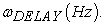All of those parameters were customized especially with the purpose to represent the same samples in the study 7.

3.2. Experimental Validation

The following tables present the calculated resonance frequenciesfrom the three beam specimens for both motions. The computational results obtained in the present work (Comput.) can be compared with the theoretical (Theo.) and experimental (Exp.) ones from the work Urgueira 7 respectively. The relative error (Error) between the computational results and the experimental data from 7 is also presented here. The reference for the relative error calculation is done neglecting the extension of the central line effect (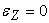) for in-plane vibrations.

Each beam specimen studied is accompanied by four graphs where the FRF are represented, with the corresponding position in the receptance matrix for both types of motion. For this case, the FRF are obtained as accelerance where the force/acceleration relationship is illustrated below each curve.

With the results obtained for the natural frequencies represented in Table 2, Table 3 and Table 4, one can see that the computational model developed guarantees results with a high degree of accuracy, in terms of relative error values. In general, the results obtained computationally can be in agreement with the experimental ones in a perspective of existing results with a relative error lower than 1% and never exceeding 10.2% for ranges up to 3000 Hz.

When comparing the results obtained for both motions, it was found that the relative error is greater in the out-of-plane case, for the three beams tested. This motion had a higher error possibly due to the cross-sectional geometry, more specifically to factors due to the relationship between the torsional stiffness and flexural stiffness modules.

Beam 1 is the one with higher average relative error values in both cases when compared to the other beams. However, the results appear to improve for a beam of identical length with an opening angle of 180°, that is, Beam 2.

The best results for the calculated error of the 3 beams under study are those of Beam 3. It was found that changing the cross-section of Beam 2 to a circular shape (Beam 3) produced an improvement in results, probably due to the increase in the torsional stiffness/flexion ratio.

• Figure 5. Beam 1 In and Out-of-Plane FRF
• Figure 6. Beam 2 In and Out-of-Plane FRF

Regarding the frequency response functions, these curves were obtained for each of the three reference beams in the form of four different FRF accelerations, allowing the comparison between them both in terms of resonance frequencies and the range of motion.

Looking at the four FRFs, it can be confirmed that in all cases, for each beam example, all of them present positive peaks at the same frequency, thus there is this proof that the computational model is consistent in the calculation of direct and indirect FRFs. The specified frequency range can be refined with such a low delay so that is possible to display a defined curve.

In a particular observation of initial values up to approximately 100 Hz, in some FRF there are very slight discontinuities that may possibly be associated with the solution in form 6 of the work Urgueira 7 that were not included in the program algorithm, due to its rare occurrence in the calculation of the roots of the characteristic polynomial.

• Figure 7. Beam 3 In and Out-of-Plane FRF

However, the appearance of the curves is clear and coherent in its domain generally, with no calculation flaws and applied to these three beams, its stable calculation range was up to nine natural frequencies.

4. The Family of Modes Concept

Once the values of natural frequencies are calculated and compared with experimental values, in the previous section, there were taken positive conclusions regarding the coherence of the obtained results. Therefore, is plausible to proceed consistently towards more complex studies such as the curves here designated as mode families, in which the consistency on calculating accurate natural frequencies is mandatory.

The program was developed with the purpose of obtaining the frequency response of a curved beam of homogeneous material and constant geometric parameters. However, the code was upgraded so that it was possible to have another type of study aiming to display the first four natural frequencies of a beam with a fixed length, varying its opening angle (therefore its radius of curvature) in a customized range, resulting in a representative graph of the four natural frequencies as a function of the beam curvature.

The set of points (or curve) that contains the natural frequencies related by the modal behaviour is hereby defined as families of modes. In section 4.1 and 4.2 is possible to see the results of that study applied the same rectangular cross-section such as Beam 1 and 2, keeping 0.754m of length. The section 4.3 approaches a curved beam with the mentioned parameters excepting the cross-section, changed to a circular one with 25mm of diameter.

Thus, for each angle the values of the first 4 natural frequencies of the beam of rectangular section were obtained from 0° to 360° opening angles, for the out-of-plane (Fig. 10) and for the in-plane motion either neglecting the effect of the extension of the central line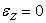(Fig. 8) or considering this effect4.1. In-Plane Vibrations

As can be following observed, the curves that represent the families of modes for the in-plane vibrations contain the first, second, third, and fourth natural frequencies, noticing that the distribution of the natural frequency points was done regularly.

As a practical example of how the opening angle changes in this type of study, observe Fig. 8 and note the beam configuration for different opening angle values.

• Figure 8. Natural Frequencies for rectangular cross-section

For the in-plane resonance frequencies, it can be seen (Fig. 8), a set of curves distributed in fairly regular ways in that their gradient on the angular domain had little variations, thus suggesting that the modes of vibration manifest with 2, 3, 4 and 5 nodes respectively along with the natural frequencies, and this is what really happens in the case of straight beams. It should be observed that these four families of modes for this type of motions seem to the behaviour expectations, namely regarding the natural frequency in which odd natural frequencies correspond to symmetrical modes and even natural frequencies correspond to antisymmetric modes, once the convergence to the straight beam, i. e. for opening angles near 0°, seems to happen without any sudden change.

This result was presented firstly because it is not as complex as the out-of-plane case, since this planar motion is easily generalized by the “law” of straight beams in terms of modal behaviour and, therefore, the work will focus more on the out-of-plane, in which case the curves have a different appearance.

• Figure 9. Extension of the central line effects εz ≠ 0 and εz = 0

The graph shown in Fig. 9 overlays the results of the natural frequency curves of the previous case with the results of the same type but accounting for the effect of the extension of the central line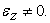The opening angle range starts at 150° and not at values close to 0°, which is justified by the fact that it is not possible to obtain results due to disturbances in the program when making calculations with radii of curvature of that order, accounting for the effects of rotatory inertia, shear deformation and effect of the extension of the central line.

Discussing the overlapping of the results, it is visible that the results are practically identical, with almost no distinction between the curves of both situations, which is in fact a sign that despite the calculation range taking into account the referred effects, the results are in accordance. However, with a closer look, a slight inflation of the results that account for the mentioned effects can be observed.

4.2. Out-of-Plane Vibrations

The aspect of the result for the out-of-plane vibrations (Fig. 10), presents different characteristics when compared to the previous result in Fig. 8. Directing the study to out-of-plane vibrations, the curves have shown changes on the gradient. Additionally, these vibrations can manifest different responses on their degrees of freedom involved, once this case has a non-planar motion. In the first case (Fig. 8) it happens that there is a dependence between these components when the motion happens because the deformation of the beam in-plane happens radially and tangentially, which implies a coupling of the displacements and what inevitably forces the rotation of the cross-section. Consequently, there is a harmony between the degrees of freedom of translation u, w, and the rotationhaving Hoppe 1 also observed this fact.

• Figure 10. Natural Frequencies for rectangular cross-section

In the out-of-plane case, there is a more complex context because the only dependence noticed is the relation on bending with the rotation of the cross-section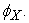Furthermore, it is not evident that a curved beam can bend due to v without rotating its section axially in x. The motion according to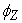(associated to torsion), is in not related to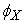but it raises the doubt if it is related to the displacement in v.

Over several studies, it can be noticed that the researchers who approached the appearance of these curves, never considered the situation of intersection between different families of modes. Consequently, the double mode subject on curved beams with specific geometries, as the ones presented here, should be considered.

4.3. Convergence to Straight Beam Model

A comparison of the results obtained by the computational model was made with the ones previously obtained experimentally and it is reasonable to assume that the model presents a good degree of reliability in the results. This reliability can be further tested by taking the study of natural frequencies literally to the limit for opening angles near to 0°, inevitably implying the convergence for a straight beam element. As a result of the extreme decrease in curvature so that the length could be preserved, it was intended to evaluate the proximity of these values to the resonance frequency values of an equivalent straight beam model calculated analytically.

In this section, the objective was to present a study where the natural frequencies of both motions were “enforced” to converge to the straight beam ones either via in-plane or out-of-plane (Fig. 11). This study is applied for a circular cross-section as Beam 3 remaining the same length (0.754m) and the transversal section diameter (25mm). Once the cross-section is symmetric on the x and y axis, the convergence for the straight beam resonance frequencies should happen for both motions as plotted in Fig. 11 and it was confirmed for extreme curvature values close to 0°.

• Figure 11. Families of modes convergence to straight beam

In Fig. 11, the results of the families of modes corresponding to in and out-of-plane, red and green respectively, are discretely plotted. The quantitative results are shown in Table 5 accompanied by the values of the first natural frequencies calculated via computational (Comput.) and analytically for the straight beam (Theo.). These four frequencies were calculated for values of opening angles decreasingly closer to 0, starting at 10°, reducing to half 5°, to 1°, and finally to 0.1°.

It is verified that the families of modes of both types of motions tend converge to the same values decreasing the opening angle (increasing the radius of curvature) to a value that, a priori would be called the natural frequency of a straight beam. It was noticed that the convergence happens at different ''rhythms''. As the convergence to the first natural frequency is happening later relatively to the others, which can be seen when comparing with the other natural frequencies that start their convergence by decreasing values from 50° approximately.

It is possible to verify that decreasing the opening angle, the values decrease their relative error in relation to the value of the theoretical natural frequency generally. The exception is the first natural frequency for 0.1° where the curvature radius achieves 432m being this way difficult the inversion of matrixes in the numerical calculation, explaining perhaps this error increment relatively to 1°. Observing the variation of this error with the decrement of the opening angle, it was not very accentuated for the 3 last natural frequencies as it was for the first one. These values of error indicate that the approximation of the computational model values to the theoretical values of the straight beam are most evident for each angle reduction value.

5. Results and Comparisons

As calculated in the work 8 the theory of Wang et al. 6 obtained different results in these curves due to the fact that it excluded the effect of rotatory inertia. Observing Fig. 12, it should be noted that the difference between considering the mentioned effect and neglecting it, brings deviations for higher natural frequencies and lower angles.

However, the representation of the families of modes, as seen in Fig. 12, was different from that which is currently obtained in the present work with the computational model. Both graphs had a similar qualitative aspect after 100° just diverging on the crossing curves question changing the natural frequencies order.

In the same way, in 2006, Tufekçi and Dogruer 12 presented a work dealing with the exact solution for the out-of-plane vibrations of a circular arch with a uniform cross-section, by taking into account the effects of shear deformation and the rotary inertia. In that work, the authors calculated frequency coefficients for the first five modes and for different opening angles for different slenderness ratios. They have discussed the phenomena of the transition of modes from torsional into flexural, by stating that this is characterized by a sharp increment in resonance frequencies of modes that occurs at a certain combination of curvature and length of the arch.

In Fig. 7 shown in that work 4 presented here, (Fig. 13 Tufekçi and Dogruer 12), it can be seen the set of the first five frequency coefficients for different opening angles, for the particular case of a free-free supported arch with a slenderness ratio of 50.

In the present work, those frequency coefficients have been re-calculated, at this time from 12 to 135 discrete values of the opening angle in Fig. 13 (Present Work). This gives a more refined distribution of those values and led to a different interpretation of the set of points that should be taken in each curve.

As it was previously discussed, these frequency coefficients tend to be distributed according to the family of modes rather than to their ordinal appearance as the in-plane vibrations. This phenomenon is also discussed in the work presented by Lee et al. 14.

• Figure 12. Out of plane family of modes comparison
• Figure 13. Frequency Coefficients comparison for slenderness ratio ʎ = 50

It was shown in this new graph of Fig. 13 (Present Work) that, instead of a sudden change in the natural frequency, there is a swap on the order of the modes. For instance, at particular angles there is an interception between two curves that would represent two distinct families of modes as it happens in Figure 12 (Present Work). The question about the sudden change on the natural frequency at that angle was clarified in Fig. 13 (Present Work), whereby one can see that there is a change in the order of the mode rather than in its natural frequency. These curves will evolve for the ones presented in Fig. 14, if one takes a different slenderness of 25, which corresponds to the case of the actual tested specimen with an angle of 180°, Beam 2. The computational model was once more reprogrammed in order to obtain the Frequency Coefficient studied in 12 having the confirmation that the alteration of the order really exists after certain angle values.

• Figure 14. Frequency Coefficients for a slenderness ratio ʎ = 25

6. Conclusions

A computational program has been implemented to calculate the in and out-of-plane response of a curved beam that considers the shear deformation and rotary inertia effects, based on an analytical model previously developed. This computational tool allows the calculation of FRF curves for customized curved beams according to the input parameters and makes possible the graphical representation of the natural frequencies for a certain range of the angles of aperture combined with the frequency interval. The main focus was to generate new results using the same references as many specialized authors used and contest them about concrete issues regarding the dynamic response of curved beams.

The results obtained can be considered accurate after the comparison with theoretical and experimental via for dynamic responses of curved beams. From the frequency response function FRF, the resonance frequencies were identified and presented as reference for specific beam cases. With the resonance frequencies, the families of modes can be represented and each of these curves is assumed to specific modal behaviours.

For the in-plane vibrations, the extension of the central line effect had not a significant influence on the studied case. The consideration of its effect led, in fact, to major deviations relatively to the experimental results and the consideration of this effect would have the consequence of unstable results during the calculations either for reduced angles or high curvature radii. Consequently, it is reasonable to neglect the mentioned effect in order to gain computational efficiency.

Once those results correspond to the straight beam model in terms of a regular and continuous convergence for the most complex values (where the radius increases drastically for very low opening angles), it can be assumed consistency on the rest of the calculations since the computational cost decreases significantly.

The out-of-plane vibrations shall be interpreted by another perspective once those curves indicate a change with the angle leading to intersection points. The change of the evolution of these families of modes could be possibly related to the flexural and torsional components. The flexural component would be predominant for lower angles and as long it increases, the torsional component would get more relevance.

The question of this swap is clarified either by performing the families of modes curves or by frequency coefficients as shown (Fig 12, 13, and 14). For years, many authors stated that those curves would have changes due to the flexural or torsional components but never considering a swap of the resonance frequencies order. For certain angle values, the phenomena of double mode should be considered. The present study should also encourage researchers for further investigation regarding the analytical solution for such curves and as well investigating the vibration modes according to the crossing points discussed, where there is a strong evidence of a double mode presence.

The current question about the dependency of the natural frequencies with the opening angle was clarified accurately with a high set of discrete values aiming to give the most approximated curves to its real appearance. The lack of points on previews works led to different interpretations which can be hereby well answered.

As it was observed, results were obtained from a computational analysis with precision in the scope of engineering applications. However, the validity of the computational model is not accurately defined, namely regarding the slenderness and R/Alt ratios as it is known that for high values of this last ratio the tendency arises of the appearance of numerical errors concretely in the inversion of the matrices (singularity problems) on the calculations.

From this study has resulted new contributions and references for researchers to move the topic of curved beams for further discussions and motivating new studies on the field of structural mechanics.

Acknowledgements

This research did not receive any specific grant from funding agencies in the public, commercial, or not-for-profit sectors.

References

  V. H. R. Hoppe, Vibrationen eines Ringes in seiner Ebene, 1871 (73) (1871) 158-170. In article View Article  A. E. H. Love, A Treatise on the Mathematical Theory of Elasticity, Dover Publications, 1944. In article  Seidel, B. S. and E. A. Erdélyi, On the vibrations of a thick ring in its own plane, J. Eng. Ind., 86 (3) (1964) 240-244. In article View Article  Rao S. S. and V. Sundararajan, In plane flexural vibrations of circular rings, ASME J. App Mech, 36 (3) (1969) 620-625. In article View Article  Timoshenko S. and D. H. Young and W. Weaver, Vibrations Problems in Engineering, John Wiley & Sons, 1974. In article  Wang T. M. and A. Lasky and M. Ahmad, Natural Frequencies for out-of-plane Vibrations of Continuous Curved Beams, Int J. Sol Struct, 20 (3) (1984) 257-263. In article View Article  A. P. V. Urgueira, Análise Dinâmica de Vigas Curvas – Desenvolvimento de um Modelo Analítico, Instituto Superior Técnico, Lisboa, Portugal, 1985. In article  Montalvão e Silva, J. M. and A. P. V. Urgueira, Out of Plane Dynamic Response of Curved Beams – An Analytical Model, Int. J. Sol Struct., 24 (3) (1988) 271-284. In article View Article  Tufekçi, E. and A. Arpaci, Exact Solution of In-Plane Vibrations of Circular Arches with Account Taken of Axial Extension, Transverse Shear and Rotatory Inertia Effects, J. Sound Vib. 209 (5), (1998) 845-856. In article View Article  Tufekçi, E. and O. Y. Dogruer, Exact Solution of Out Of Plane Free Vibrations of a Circular Beam with Uniform Cross Section, Faculty of Mechanical Engineering Istanbul Technical University, Istanbul, Turkey, 2002. In article View Article  Tufekçi, E., T. Bostanci, O. Ozdemirci, and O. Oldac, Experimental Verification of Numerical and Analytical Solutions of Free Vibrations of Curved Beams, Faculty of Mechanical Engineering Istanbul Technical University, Istanbul, Turkey, 2006. In article  Tufekçi, E. and Dogruer O. Y., Out-of-plane free vibration of a circular arch with uniform cross-section: Exact solution, J. Sound Vib. 291 (3-5) (2006) 525-538. In article View Article  Raposo, F. O. Análise Dinâmica de Vigas Curvas – Desenvolvimento de um Programa Computacional, Faculdade de Ciências e Tecnologia – Universidade Nova de Lisboa, Caparica, Portugal, 2018 (in Portuguese). (https://run.unl.pt/handle/10362/56415). In article  Lee, B.K. Oh, S.J., Mo, J.M., Lee, T.E., Out-of-plane free vibrations of curved beams with variable curvature, J. Sound Vib. 318 (1-2) (2008), 227-246. In article View ArticleThis work is licensed under a Creative Commons Attribution 4.0 International License. To view a copy of this license, visit http://creativecommons.org/licenses/by/4.0/